## Proof without words: The Pythagorean Theorem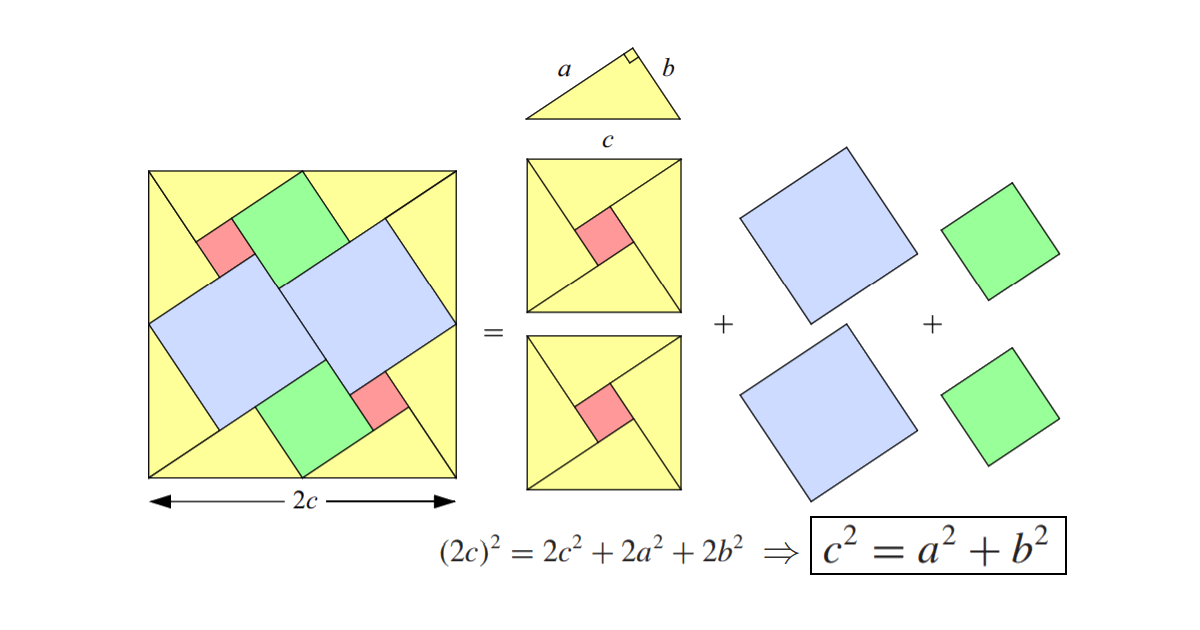### Explanation

We take a right triangle with sides $$a$$, $$b$$ and $$c$$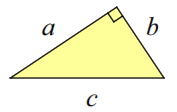Then we duplicate the yellow triangle to get 8 similar triangles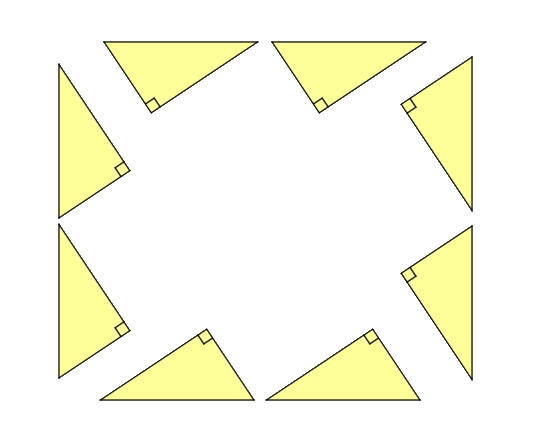Now we assemble the triangles to get the sides of a square like it is shown in the next figure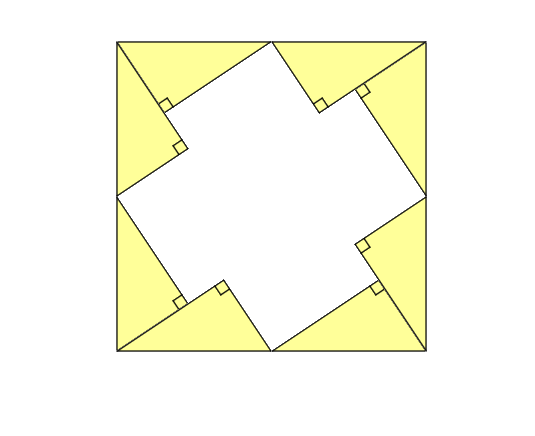Next, let’s try to fill the white space until we get a filled square.  We start by adding 2 blue squares of the same side within the previous figure. We get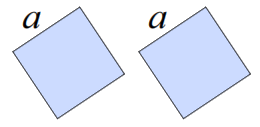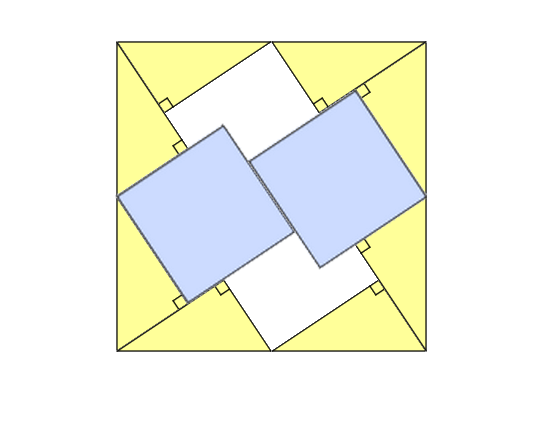Then, we add 2 green squares of side b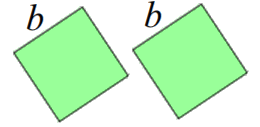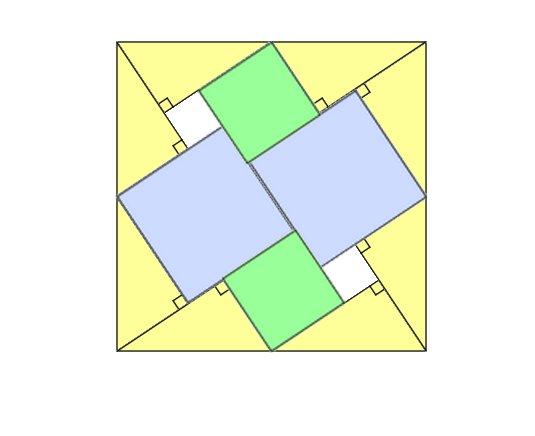Finally, we fill the rest by adding the two last squares of the same side coloured in red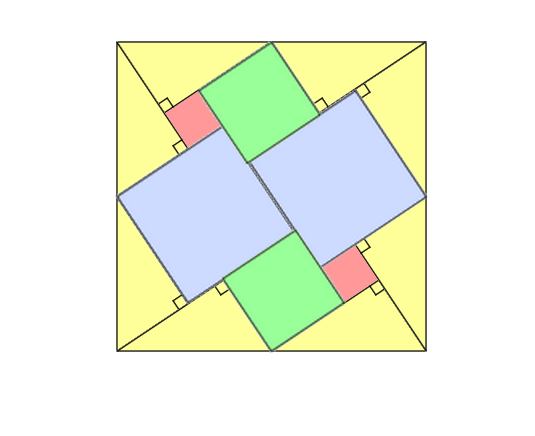We can divide the area of the biggest square into these parts to get our final result.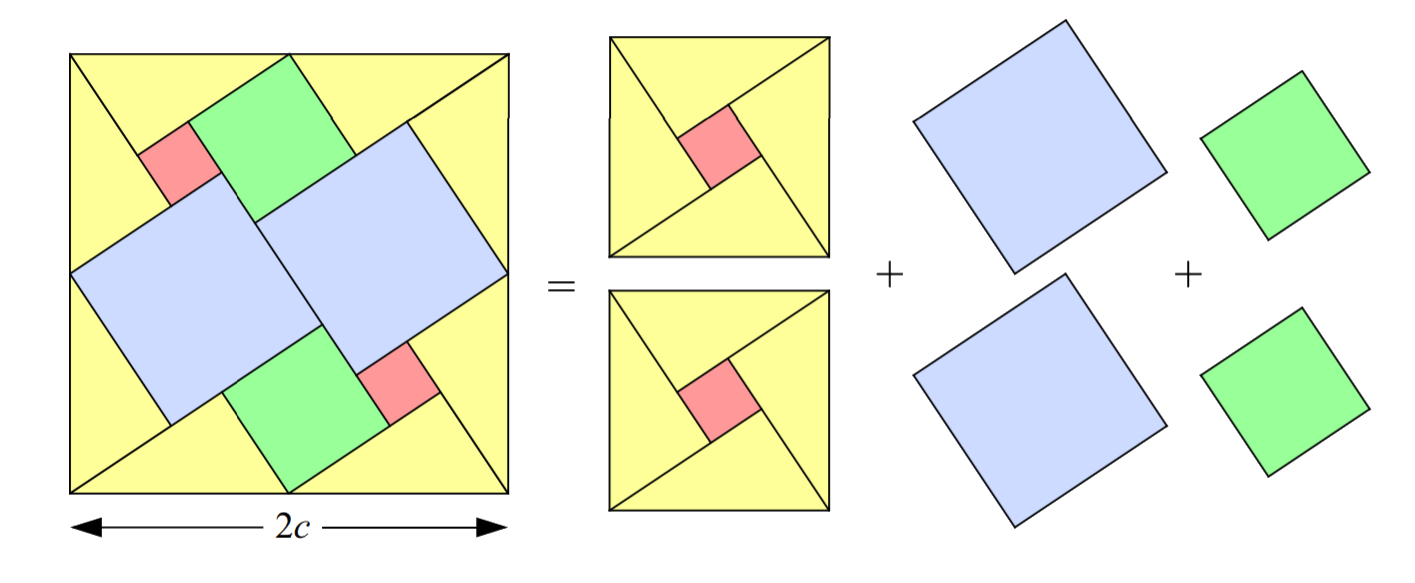$\huge \left(2c\right)^{2}=2c^{2}+2a^{2}+2b^{2}\Rightarrow c^{2}=a^{2}+b^{2}$